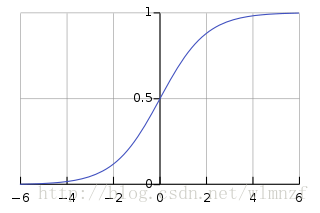PS:本文中的log等同于我们国内的ln

### sigmoid函数

之前那一文中提到了一般的梯度上升的公式推导，但是在《机器学习实战》一书中，实现的是分类方法，因此，虽然最终的结果相似，但是其实本质有很大的不同。

一般来讲我们把实物分成两类，因此我们需要将结果映射到两个结果(是或非)，因为一般的阶跃函数在求导之类的问题上会变得相当复杂，因此我们用一个更加圆滑的sigmoid函数来映射，所有输入到这个函数的实数都会被转化到0-1之间，它的公式为 $g(z)=\frac{1}{1+e^{-z}}$

同时它对应的图像如图所示:于是我们可以将得到的结果进行四舍五入，分类成0或1

### Logistic 回归

这里的意思是，将我们的分类边界线作模型，进行拟合，并以此来分类。

我们假设经过sigmoid函数处理过的结果为 $h_{\Theta}(x)$ ,因为是在0-1之间，因此可以看做是概率，另外，我们可以假设，分类到0或者1的概率。

$$P(y=1|x;\theta)=h_{\theta}(x) \\ P(y=0|x;\theta)=1-h_{\theta}(x) \tag{1}$$

$$p(y|x;\theta)=(h_\theta(x))^y(1-h_\theta(x))^{1-y} \tag{2} \\$$

### 梯度上升解决回归问题

#### 1. 最大似然估计

这里我们使用最大似然估计法，这个在大学的高等数学中应该都有学习过，就不在赘述。这里假设我们有m个训练集。

$$L( \theta )=\prod_{i=1}^{m}p(y^{(i)}|x^{(i)};\theta)=\prod_{i=1}^{m}(h_\theta(x^{(i)}))^{y^{(i)}}(1-h_\theta(x^{(i)}))^{1-{y^{(i)}}} \tag{3}$$

为了求导方便，我们一般会将似然函数L加上log函数，因为log函数是递增函数，因此不影响似然函数求最值。

$$l(\theta)=logL(\theta)=\sum_{i=1}^my^{(i)}logh(x^{(i)})+(1-y^{(i)})log(1-h(x^{(i)})) \tag{4}$$

将l函数对$\theta$求导

$$\frac{\partial}{\partial\theta_j }l(\theta)=(y\frac{1}{h_\theta (x)}-(1-y)\frac{1}{1-h_\theta (x)})\frac{\partial}{\partial\theta_j}h_\theta x \tag{5}$$

#### 2. sigmoid函数求导

$$\begin{equation} \begin{split} &h’(x)=\frac{d}{dx}\frac{1}{1+e^{-x}}\\ &=\frac{1}{(1+e^{-x})^2} (e^{-x})\\ &=\frac{1}{(1+e^{-x})}(1-\frac{1}{(1+e^{-x})})\\ &=h(x)(1-h(x)) \end{split} \end{equation} \tag{6}$$

#### 3. 似然估计后续

从上一篇文章，或者从《机器学习实战》chapter5 中可得sigmoid函数h(x)的输入函数是$w=\theta^Tx$,将其代入公式(4)，得到

$$\begin{equation} \begin{split} &l’(\theta)=(y\frac{1}{h(\theta^Tx)}-(1-y)\frac{1}{1-h{(\theta^Tx)}}) \frac{\partial}{\partial\theta_j}h(\theta^Tx)\\ &=(\frac{1}{h(\theta^Tx)}-(1-y)\frac{1}{1-h(\theta^Tx)})h(\theta^Tx)(1-h(\theta^Tx)\frac{\partial}{\partial_j}\theta^Tx)\\ &=(y(1-h(\theta^Tx))-(1-y)h(\theta^T x))x_j\\ &=(y-h_\theta(x))x_j \end{split} \end{equation} \tag{7}$$

William

wlmnzf

IIE
CAS
csuncleWelcome！
I'm happy it's useful to you!